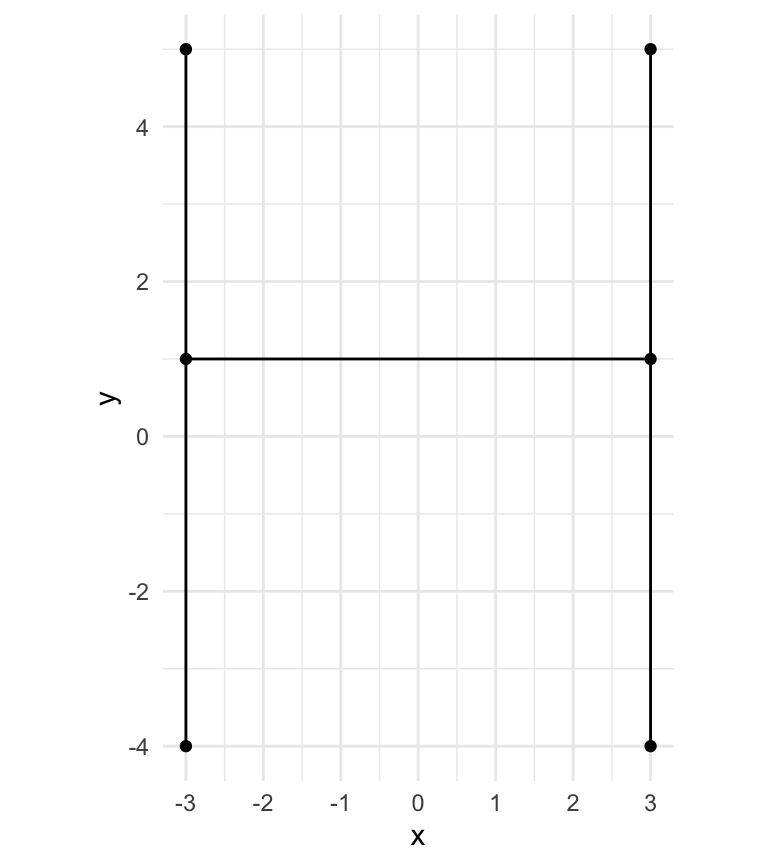library(hershey)
library(ggplot2)

## JHF - Hershey Font File format

The Hershey Font File format (.jhf) is a text file with encodings for all the glyphs in the font.

The text is hard-wrapped at 72 characters-per-line, so longer glyphs get split across multiple lines.

## Glyph Encoding

The following is from Paul Bourke’s dataformats page.

The structure is basically as follows:

Position Interpretation
0-4 Identifier (integer) - not unique!
5-7 Number of vertices (integer)
8 Left position
9 Right position
Every pair of characters represents a vertex

All vertex coordinates are given as a pair of characters. The numeric value of each vertex its ASCII value relative to the ASCII value of ‘R’.

The vertex coordinate of " R" indicates a pen up operation i.e. the drawing pen moves to the next location, but does not draw a stroke.

As an example consider the glyph: 8 9MWOMOV RUMUV ROQUQ

• ID = 8
• Number of vertices = 9 - this means there are a total of 2*9 = 18 characters to decode.
• Left position = ‘M’ (ASCII = 77). Relative to ‘R’ (ASCII = 82), this is -5
• Right position = ‘W’ (ASCII = 87). Relative to ‘R’ = +5
• First coordinate is “OM” = (-3,-5)
• Second coordinate is “OV” = (-3,4)
• Raise the pen " R"
• Move to “UM” = (3,-5)
• Draw to “UV” = (3,4)
• Raise the pen " R"
• Move to “OQ” = (-3,-1)
• Draw to “UQ” = (3,-1)

This glyph string can be converted to a data.frame (using hershey::convert_glyph_to_df()) and plotted with ggplot.

glyph    <- '    8  9MWOMOV RUMUV ROQUQ'
glyph_df <- hershey::convert_glyph_to_df(glyph)

glyph_df
#>    x  y left right width stroke idx
#> 1 -3  5   -5     5    10      0   1
#> 2 -3 -4   -5     5    10      0   2
#> 4  3  5   -5     5    10      1   3
#> 5  3 -4   -5     5    10      1   4
#> 7 -3  1   -5     5    10      2   5
#> 8  3  1   -5     5    10      2   6

ggplot(glyph_df, aes(x, y, group = stroke)) +
geom_point() +
geom_path() +
coord_equal() +
theme_minimal() +
scale_x_continuous(breaks = scales::pretty_breaks()) +
scale_y_continuous(breaks = scales::pretty_breaks())